peteroznewman
Subscriber

From Wikipedia, Absolute thermal resistance is the temperature difference across a structure when a unit of heat energy flows through it in unit time.

So R = (Ths - Tamb)/Q  where R is Thermal Resistance in units of K/W, Q is heat flow in W and (Ths - Tamb) is the change in temperature in Kelvin across the thermal boundary layer, where Ths is the hot surface temperature and Tamb is the ambient temperature.

This could be written

Q = (Ths - Tamb)/R

ANSYS implements convective heat transfer using a film coefficient h in units or W/K.m^2 and that is applied to a surface. ANSYS calculates the area of that surface to know a value for A.

ANSYS will calculate Q using the equation Q = hA(Ths - Tamb)

Therefore 1/R = hA or as you said, h = 1/(R*A)

If your Area comes from SpaceClaim without units as P7, you can add units as shown in P9.  Note that P8 is coming from Mechanical from the Convective boundary condition.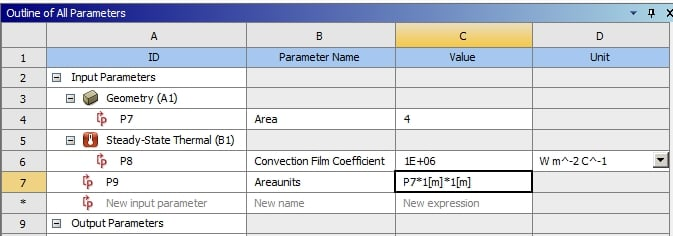Let's say the value of R will be entered as an input. In this example, R = 7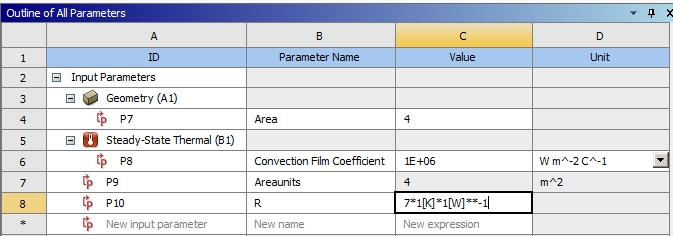Now you can type the equation for h.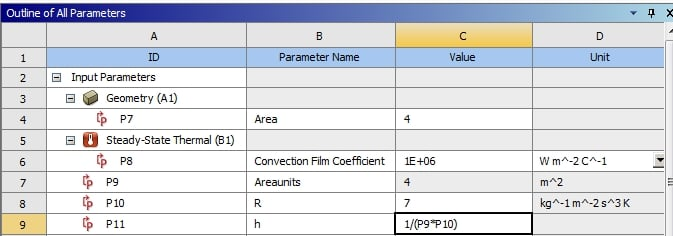Edit the Properties of P8, which came from Mechanical Convective boundary condition.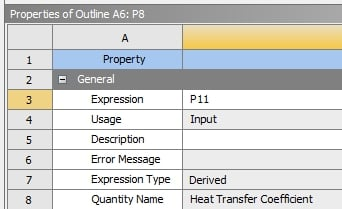Now the value of h used in the Convective BC is calculated from R and A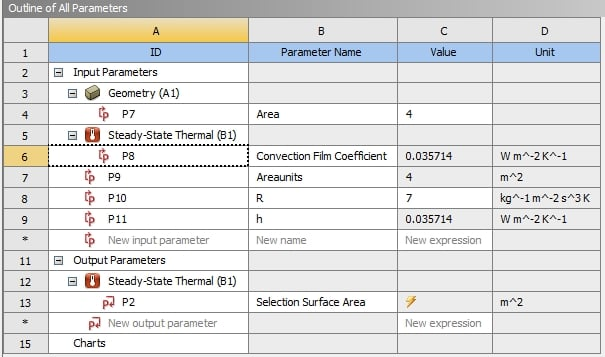Type in a range of R values and Areas in the Parameter Set table.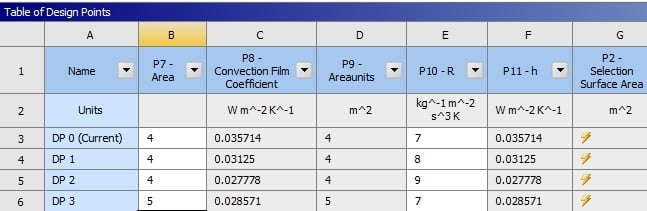P2 is an output from Mechanical measuring a specific Named Selection Face in the model.